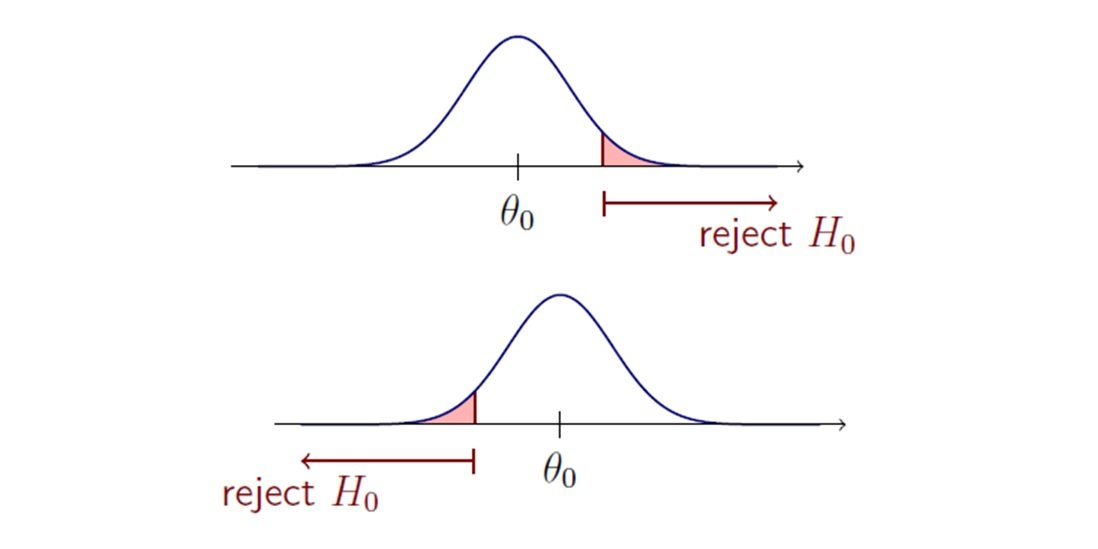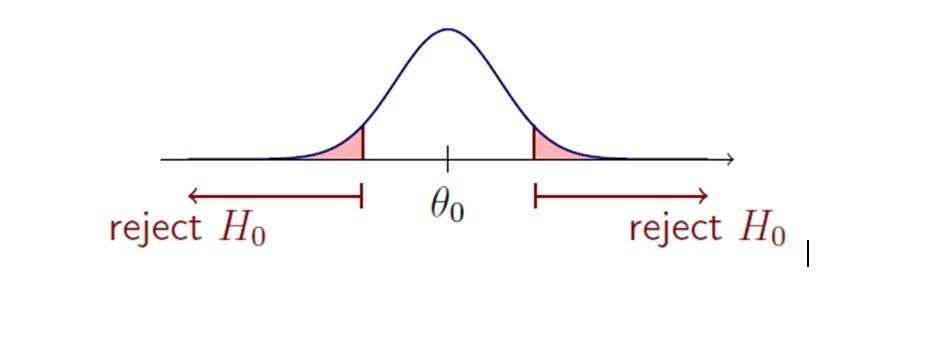Hypothesis Testing

# Hypothesis Testing Assignment Help

Home / Statistics Assignment Help / Hypothesis Testing

# Hypothesis testing

Hypothesis tests can be one sided or two sided tests as explained below.

One tailed tests are directional in nature:

H0: µ1 - μ2 ≤ 0
H1: μ1 - μ2 > 0

This is a one-tailed test in which the critical region lies in the right-tail of the test statistic.Two tailed tests are not directional:

H0: μ1 - μ2 = 0
H1: μ1 - μ2 ≠ 0## Significance of P-value:

P-value ≤ α ⇒ Reject H0 at level α
P-value > α ⇒ Do not reject H0 at level α

## When to Reject H0

Denoting our subject probability as follows:

Level of significance, α:It is defined before doing hypothesis test and is used to decide rejection region
Rejection region: Region outside the confidence interval as shown below.## When to use what?

 Comparison of means Parametric Tests Non-parametric Tests Differences between the means of two independent groups Independent t-test Mann-Whitney test Differences between paired (matched) samples e.g. weight before and after a diet for each subject Paired t-test Wilcoxon signed rank test Differences in the means of 3+ independent groups for one variable One-way ANOVA One-way ANOVA Differences between 3+ measurements on the same subject Repeated Measures ANOVA Friedman test Strength of a relationship between 2 continuous variables Pearson's Correlation Co-efficient Spearman's Correlation co-efficient Predicting the value of one variable given the value of a predictor variable Simple Linear Regression Assessing the relationship between two categorical variables. Chi-squared test

### Submit Homework

Submit your homework for a free quote